# (a) Let h() be defined by 1. h(x) h2, х > а where h h2 and a are constants. Find the first and second derivatives of...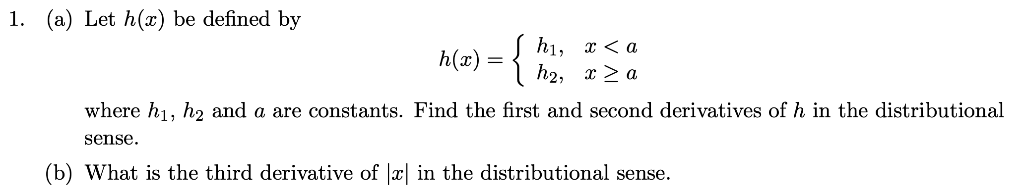(a) Let h() be defined by 1. h(x) h2, х > а where h h2 and a are constants. Find the first and second derivatives of h in the distributional sense (b) What is the third derivative of |x| in the distributional sense.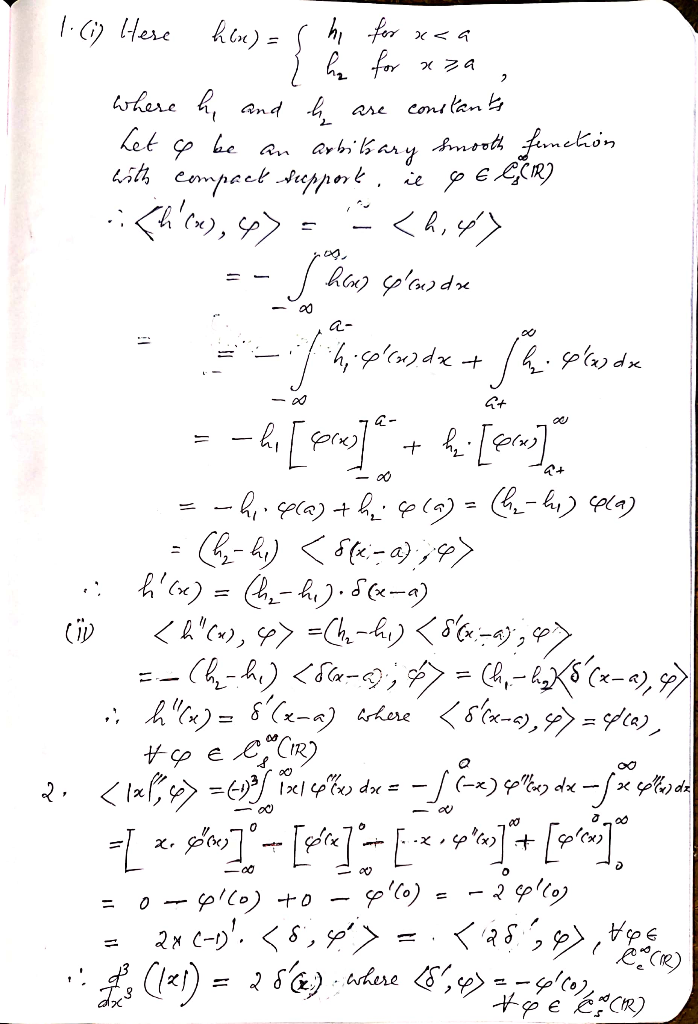##### Add Answer of: (a) Let h() be defined by 1. h(x) h2, х > а where h h2 and a are constants. Find the first and second derivatives of...
Similar Homework Help Questions
• ### 1. Consider the distribution Tylo]-)dx where f(x)H(x), and H() is the Heavi- side function. Find ...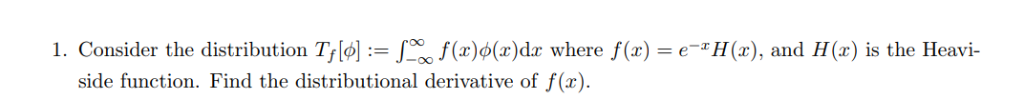1. Consider the distribution Tylo]-)dx where f(x)H(x), and H() is the Heavi- side function. Find the distributional derivative of f (r 1. Consider the distribution Tylo]-)dx where f(x)H(x), and H() is the Heavi- side function. Find the distributional derivative of f (r

• ### Estimate the second derivative of the following function using stencils for the FORWARD and CENTRAL derivatives for an order of accuracy of O(h2) for each. Use a step size of h -1. fo)x-2x2...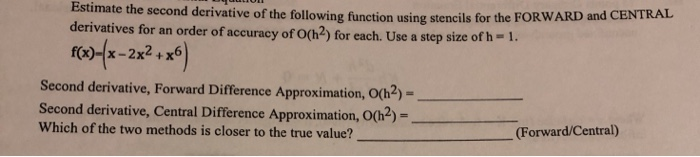Estimate the second derivative of the following function using stencils for the FORWARD and CENTRAL derivatives for an order of accuracy of O(h2) for each. Use a step size of h -1. fo)x-2x2 +6 Second derivative, Forward Difference Approximation, o(h2)- Second derivative, Central Difference Approximation, O(h2) Which of the two methods is closer to the true value? (Forward/Central 12.5 points Differential Equation Estimate the second derivative of the following function using stencils for the FORWARD an derivatives for an order...

• ### Let X and Y have the joint pmf defined by (х, у) (1,2) (0,0) (0,1) (0,2)...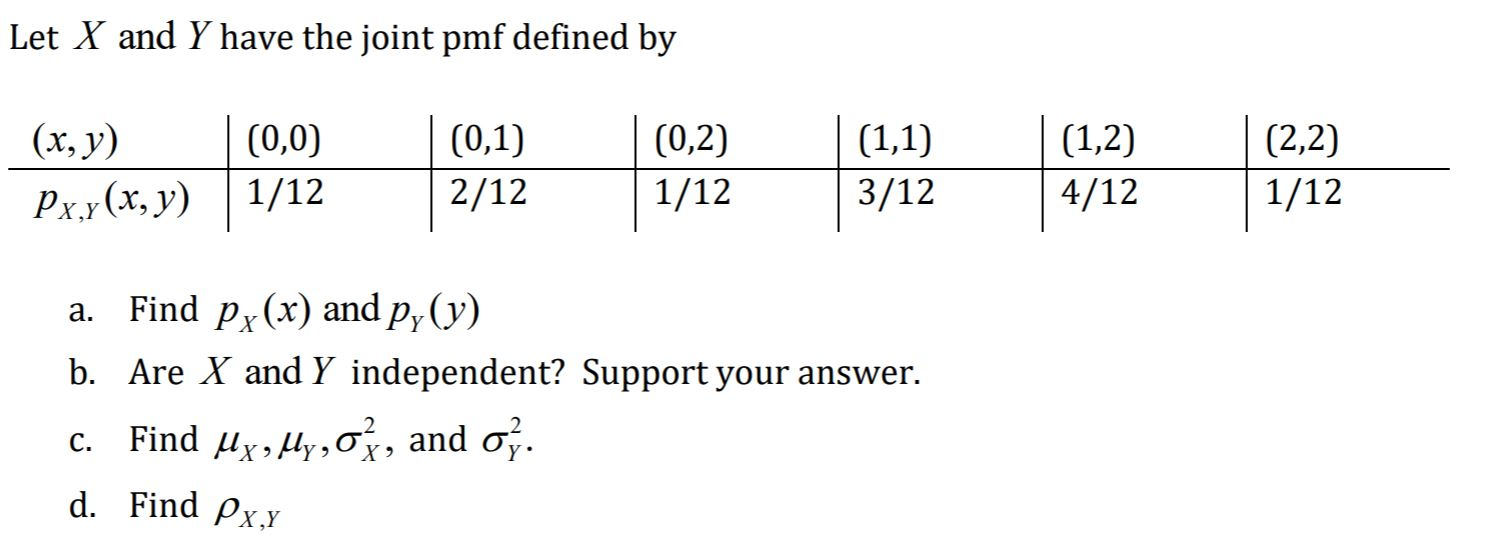Let X and Y have the joint pmf defined by (х, у) (1,2) (0,0) (0,1) (0,2) (1,1) (2,2) 2/12 1/12 3/12 1/12 1/12 4/12 Pxy (x, y) Find py (x) and p, (y) а. b. Are X and Y independent? Support your answer. Find x,y,, and o, С. d. Find Px.Y

• ### Given a second order linear homogeneous differential equation а2(х)у" + а (х)У + аo(х)у — 0...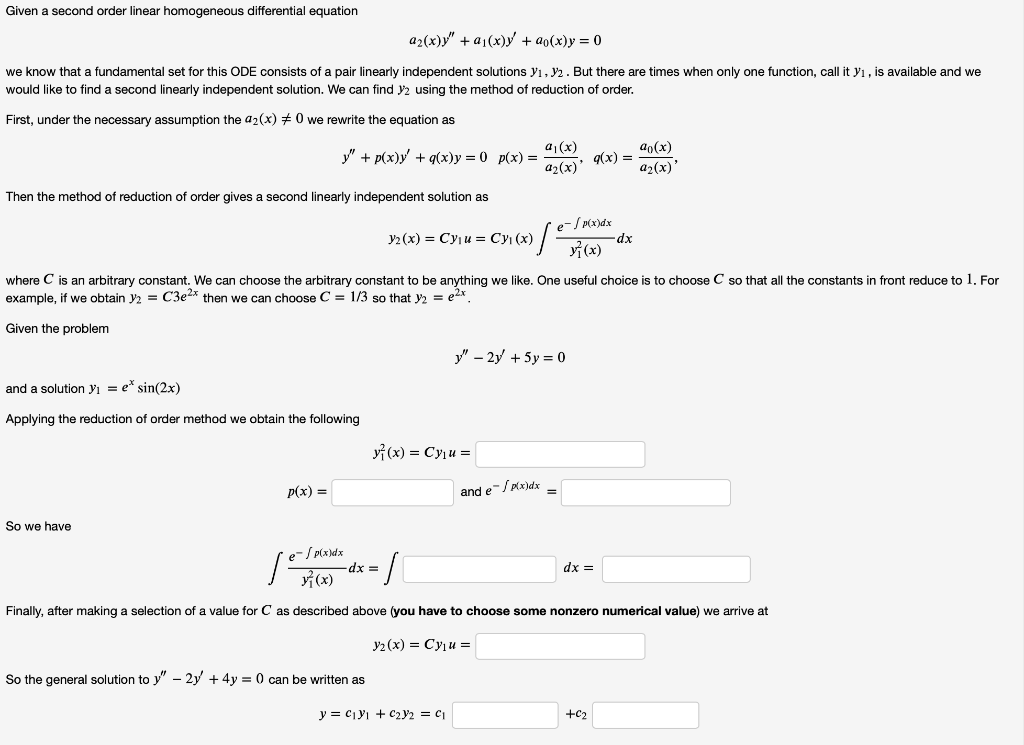Given a second order linear homogeneous differential equation а2(х)у" + а (х)У + аo(х)у — 0 we know that a fundamental set for this ODE consists of a pair linearly independent solutions yı, V2. But there are times when only one function, call it y, is available and we would like to find a second linearly independent solution. We can find y2 using the method of reduction of order. First, under the necessary assumption the a2(x) F 0 we rewrite...

• ### Consider the standard second order finite difference approximation to the second derivative h2 Let;") =e.mjh for , = 1, M-1.h=r/M. (b) Prove that the farthest eigenvalue from the origin approach...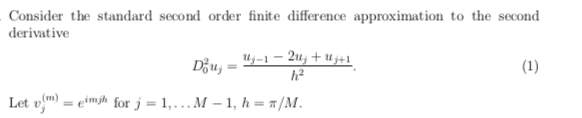Consider the standard second order finite difference approximation to the second derivative h2 Let;") =e.mjh for , = 1, M-1.h=r/M. (b) Prove that the farthest eigenvalue from the origin approaches -/h2 as h0 and use this to explain why the ODE systenm du(t) is stiff Consider the standard second order finite difference approximation to the second derivative h2 Let;") =e.mjh for , = 1, M-1.h=r/M. (b) Prove that the farthest eigenvalue from the origin approaches -/h2 as h0 and use...

• ### 5. Find the derivative matrices of the following composition of functions. (а) fog where f (x,...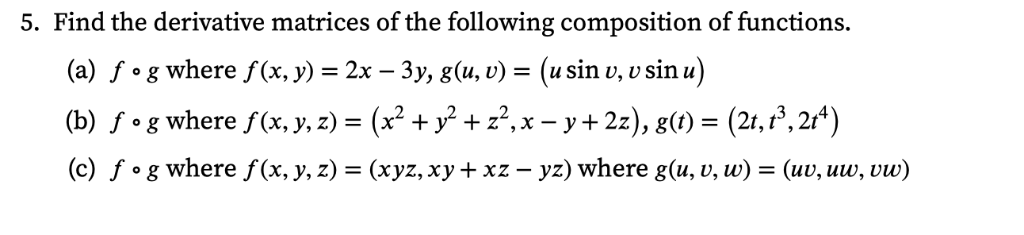5. Find the derivative matrices of the following composition of functions. (а) fog where f (x, у) — 2х — 3у, g(u, v) - (usin u, U sin u) (Ъ) f.g where f(х, у, 2) %3D (x? + у? +2?,х— у+2:), g() %3 (2, 13, 2/4) (с) fog wherе f (x, у, z) 3D (хуz, ху + xz — yz) where g(u, v, w) %3D (uu, uw, vw) 5. Find the derivative matrices of the following composition of functions. (а)...

• ### 4. For f(x) = e-* and h = 0.10 where, C = 1.** a) Use centered...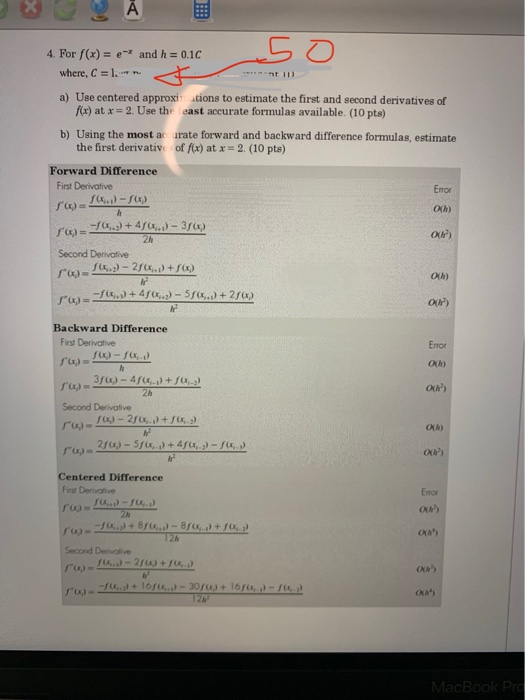4. For f(x) = e-* and h = 0.10 where, C = 1.** a) Use centered approximations to estimate the first and second derivatives of f(x) at x = 2. Use the east accurate formulas available. (10 pts) b) Using the most acurate forward and backward difference formulas, estimate the first derivative of f(x) at x 2. (10 pts) Forward Difference First Derivative 7.) - SD Error OM or) = -1.) + 40..) - 3 ) 2h Second Derivative 'w...

• ### Approximating derivatives Forward difference f(x) - f(a - h) f, (x) f, (x) (x) (8. f)(x)= (df)(x)...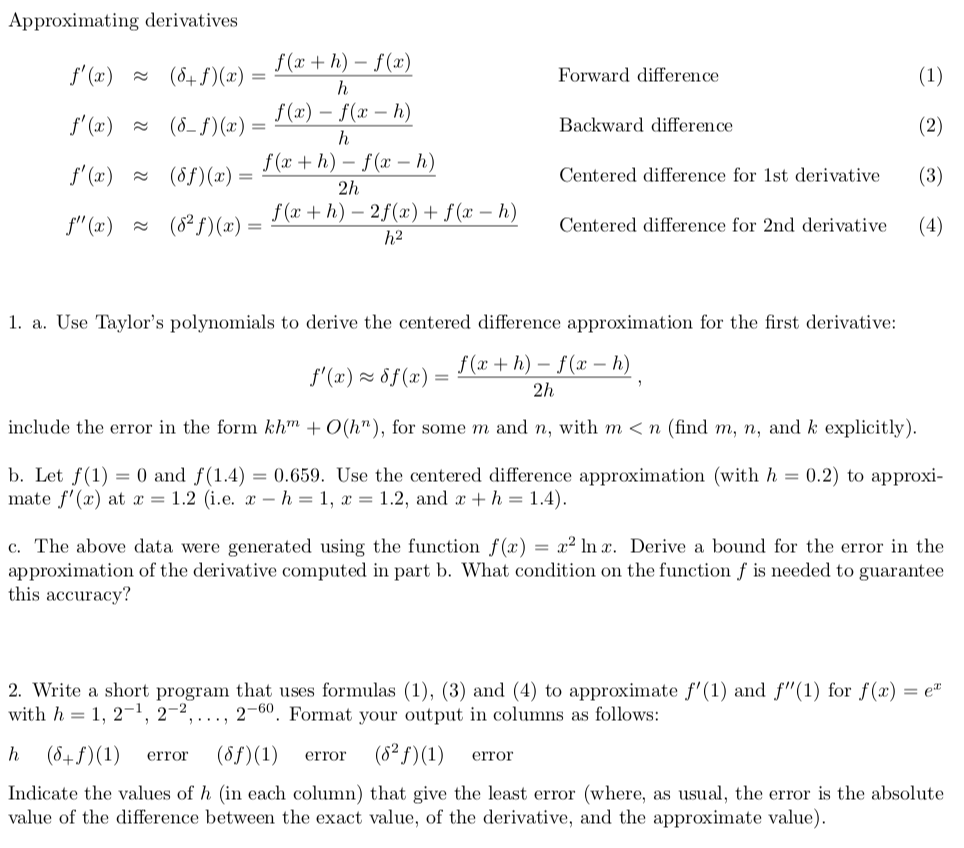Please help with this question. Thank you. Approximating derivatives Forward difference f(x) - f(a - h) f, (x) f, (x) (x) (8. f)(x)= (df)(x)=f(x+h)-f(x-h) (62 f)(x)- ~ Backward difference ~ Centered difference for 1st derivative (3) fa-h h2 Centered difference for 2nd derivative s 1. a. Use Taylor's polynomials to derive the centered difference approximation for the first derivative: h) - f(a- h include the error in the forn khTn + 0(N), for som e m and n, with m...

• ### Calculus III 1) Identify each of the following surfaces: а) z' %3x? - 5у" b) z...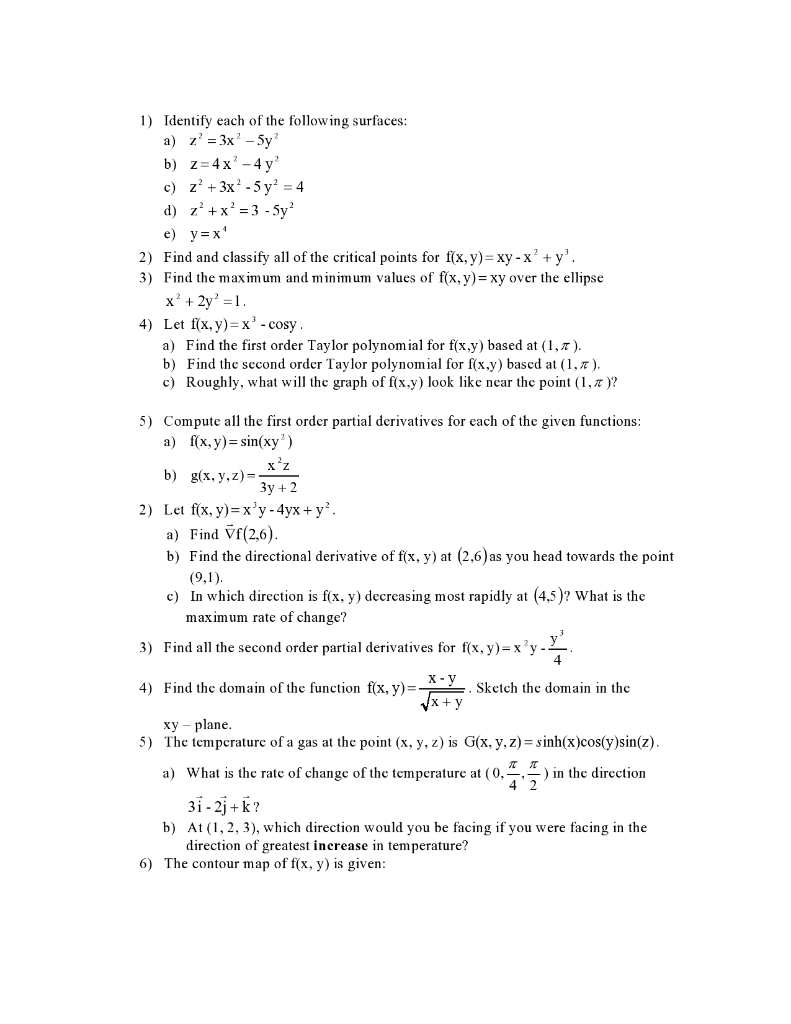Calculus III 1) Identify each of the following surfaces: а) z' %3x? - 5у" b) z 4x2-4 y c) z2+3x2-5y = 4 d) z2x23-5y e) у3х* 2) Find and classify all of the critical points for f(x, y)=xy -x2 + y'. 3) Find the maximum and minimum values of f(x,y)=xy over the ellipse х* + 2y %3D1. 4) Let fx, y) x3 -cosy a) Find the first order Taylor polynomial for f(x,y) based at (1,7). b) Find the sccond order...

• ### Where x dot means the first derivative of x, x dot dot means second derivative of...

Where x dot means the first derivative of x, x dot dot means second derivative of x. Show that the initial value problem (IVP), pfx = 1 , 2(0) = x(0) = 0, with a constant, 0 < a < 1 , has a solution of the form x(t) = bt" where b and r are constants, at least for t > 0. Find specific values for b and r, depending on a.

Need Online Homework Help?